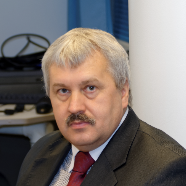# Application of the Monte Carlo algorithm for solving volume integral equation in light scattering simulations - Publication - MOST Wiedzy

## Application of the Monte Carlo algorithm for solving volume integral equation in light scattering simulations### Abstract

Various numerical methods were proposed for analysis of the light scattering phenomenon. Important group of these methods is based on solving the volume integral equation describing the light scattering process. The popular method from this group is the discrete dipole approximation (DDA). DDA uses various numerical algorithms to solve the discretized integral equation. In the recent years, the application of the Monte Carlo (MC) algorithm as one of them was proposed. In this research, we analyze application of the MC algorithm for two cases: the light scattering by large particles and by random conglomerates of small particles. We show that if proper preconditioning of the numerical problem is applied, the MC algorithm can solve the underlying systems of linear equations. We also show that the efficiency of the MC algorithm can be increased by reusing performed computations for various incident electromagnetic waves and the applicability of the MC algorithm depends on the particular use case. It is unlikely to be used in the case of light scattering by the large particles due to computational times inferior comparing with the other numerical methods but may become useful in the case of light scattering by the random conglomerates of small scattering particles.

### Citations

• 0

CrossRef

• 1

Web of Science

• 0

Scopus

### Authors (2)

•### Maciej Kraszewskimgr inż.

•### Details

Category:
Articles
Type:
artykuły w czasopismach
Published in:
OPTICA APPLICATA no. 50, pages 1 - 15,
ISSN: 0078-5466
Language:
English
Publication year:
2020
Bibliographic description:
Kraszewski M., Pluciński J.: Application of the Monte Carlo algorithm for solving volume integral equation in light scattering simulations// OPTICA APPLICATA -Vol. 50,iss. 1 (2020), s.1-15
DOI:
Digital Object Identifier (open in new tab) 10.37190/oa200101
Sources of funding:
• Działalność statusowa
Verified by:
Gdańsk University of Technology

seen 22 times

2017

2015

2016

2020